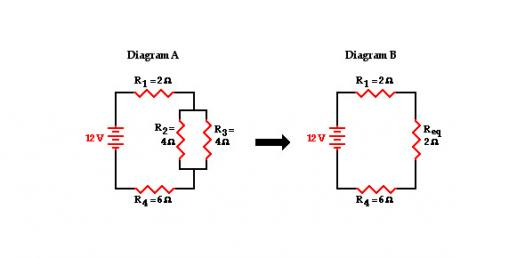# Combination Circuits

Approved & Edited by ProProfs Editorial Team
At ProProfs Quizzes, our dedicated in-house team of experts takes pride in their work. With a sharp eye for detail, they meticulously review each quiz. This ensures that every quiz, taken by over 100 million users, meets our standards of accuracy, clarity, and engagement.
M
Community Contributor
Quizzes Created: 6 | Total Attempts: 27,880
Questions: 10 | Attempts: 1,597SettingsThere are 10 questions in this part.

• 1.

### Consider the combination circuit in the diagram below. Use the diagram to answer the following questions. (Assume that the voltage drops in the wires themselves in negligibly small.)The electric potential difference (voltage drop) between points B and C is _____ (greater than, equal to, less than) the electric potential difference (voltage drop) between points J and K.

Explanation
The electric potential difference (voltage drop) between points B and C is greater than the electric potential difference (voltage drop) between points J and K because the current flows through a longer path from B to C compared to the path from J to K. The longer path results in a greater resistance, which in turn leads to a greater voltage drop between points B and C.

Rate this question:

• 2.

### The electric potential difference (voltage drop) between points B and K is _____ (greater than, equal to, less than) the electric potential difference (voltage drop) between points D and I.

Explanation
The electric potential difference (voltage drop) between points B and K is greater than the electric potential difference (voltage drop) between points D and I. This means that the amount of electric potential energy lost per unit charge is higher between points B and K compared to points D and I. This could be due to factors such as the distance between the points or the presence of a higher electric field between B and K.

Rate this question:

• 3.

### The electric potential difference (voltage drop) between points E and F is _____ (greater than, equal to, less than) the electric potential difference (voltage drop) between points G and H.

Explanation
The electric potential difference between two points is determined by the work done in moving a unit charge from one point to the other. In this case, the statement "equal to" suggests that the voltage drop between points E and F is the same as the voltage drop between points G and H. This implies that the amount of work required to move a unit charge between these two pairs of points is the same, indicating that the electric potential difference is equal in both cases.

Rate this question:

• 4.

### The electric potential difference (voltage drop) between points E and F is _____ (greater than, equal to, less than) the electric potential difference (voltage drop) between points D and I.

Explanation
The electric potential difference (voltage drop) between points E and F being equal to the electric potential difference (voltage drop) between points D and I suggests that the two pairs of points have the same electric potential difference. This means that the change in electric potential between points E and F is the same as the change in electric potential between points D and I.

Rate this question:

• 5.

### The electric potential difference (voltage drop) between points J and K is _____ (greater than, equal to, less than) the electric potential difference (voltage drop) between points D and I.

Explanation
The electric potential difference (voltage drop) between points J and K is greater than the electric potential difference (voltage drop) between points D and I. This means that there is a larger difference in electric potential between points J and K compared to points D and I. This could be due to factors such as the distance between the points, the amount of charge present, or the presence of any resistors or other components in the circuit.

Rate this question:

• 6.

### The electric potential difference between points L and A is _____ (greater than, equal to, less than) the electric potential difference (voltage drop) between points B and K.

Explanation
The electric potential difference between points L and A is equal to the electric potential difference between points B and K. This means that the amount of electric potential energy required to move a unit charge from L to A is the same as the amount required to move it from B to K. Therefore, the voltage drop between these two pairs of points is the same.

Rate this question:

• 7.

• 8.

• 9.

### Use the concept of equivalent resistance to determine the unknown resistance (in ohms) of the identified resistor that would make the circuits equivalent. Leave your answer in 2 sf.

Explanation
The unknown resistance of the identified resistor should be 18 ohms in order to make the circuits equivalent.

Rate this question:

• 10.

### Analyze the following circuit. The total current in the circuit is ____ A. Leave your answer i s 2sf.

Explanation
The total current in the circuit is 3.0 A because the current in a series circuit remains constant throughout. Since there are no branches or parallel paths in the circuit, the current flowing through each component is the same. Therefore, the total current is equal to the current flowing through any one of the components, which is 3.0 A.

Rate this question:

Related TopicsBack to top
×

Wait!
Here's an interesting quiz for you.